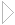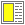Linguistic abstraction - slide 22 : 22

The eval and apply primitives

The implementation primitive eval of a Lisp systems is typically made available in the language, hereby providing access to evaluation of syntactical expressions (lists) in a given environment

The apply primitive is also available as a convenient mechanism for application of a function, in cases where all the parameters are available in a list

 Expression Value ```(let* ((ttl "My Document") (bdy (list 'p "A paragraph")) (doc (list 'html (list 'head (list 'title ttl)) (list 'body bdy))) ) (render (eval doc)))``` ``` My Document

A paragraph

``` ```(let* ((ttl "My Document") (bdy (list 'p "A paragraph")) (doc `(html (head (title ,ttl)) (body ,bdy)))) (render (eval doc)))``` ``` My Document

A paragraph

``` `(+ 1 2 3 4)` `10` `(+ (list 1 2 3 4))` ```Error: + expects argument of type number; given (1 2 3 4)``` `(apply + (list 1 2 3 4))` `10`